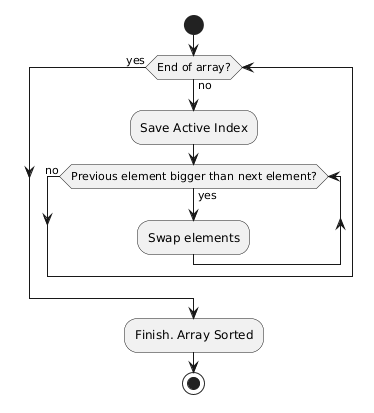Insertion Sort Algorithm

Insertion Sort appeared a long time ago. Intuitively, we use it to sort the cards in hand during card games. This is the easiest way to illustrate how this algorithm works.

How the algorithm works

Each element of the input sequence is scanned one at a time and compared with each element starting from the beginning of the list. The selected item takes its place in the list as soon as its place is found. And so with each element of the list.

The time complexity of the algorithm is O(n^2), but on small amounts of data, the algorithm sometimes performs very well.

Video shows how algorithm works

Sequence of operations

For example, let’s take the list [5, 3, 1, 6, 4].

We take the first item from the list. This is “5”. If it were a list of one element, then we would say that a list of one element is always sorted.

Moving on to the next item. This is “3”. Where should be “3” relative to “5”. Answer: before “5”. To do this, we must put “3” in front of “5”, and move “5”.

Now the list has taken the following form [3, 5, 1, 6, 4]. We can now say that the sublist [3, 5] is sorted.

Take the next item. This is “1”. Compare with the elements in front of it.
5 > 1, 3 > 1.
Obviously, “1” must come before “5” and “3”.

Let’s move the sublist [3, 5] forward and put “1” in front of the sublist.

Now the list has taken the following form [1, 3, 5, 6, 4]. We can now say that the sublist [1, 3, 5] is sorted.

Take the next item. This is “6”. Compare with the elements in front of it.
5 <6.
It makes no sense to check further since the sublist [1, 3, 5] was sorted. Item “6” remains in place.

Now the list has taken the following form [1, 3, 5, 6, 4]. We can now say that the sublist [1, 3, 5, 6] is sorted.

Take the next item. This is “4”. Compare with the elements in front of it.
6 > 4, 5 > 4, 3 < 4.
There is no point in checking further since the sublist [1, 3, 5, 6] has been sorted. Obviously, “4” must come before “6”, “5”, but before “3”.

Let’s move the sublist [5, 6] forward, and put “4” in front of the sublist.

Now the list has taken the following form [1, 3, 4, 5, 6]. We have reached the end of the list and we can say that the list is all sorted from the original list.

DiagramSample code

Below you will get a link to GitHub repo with Kotlin, Java, and Python source code